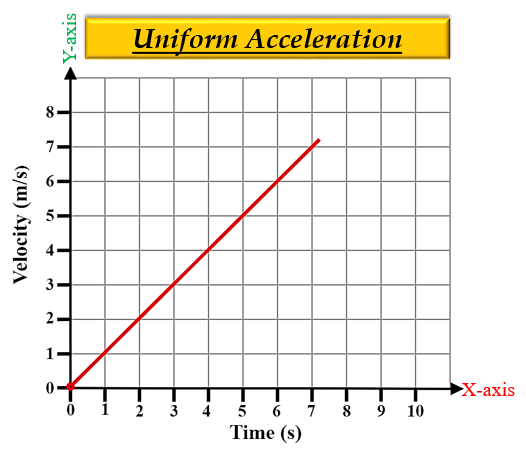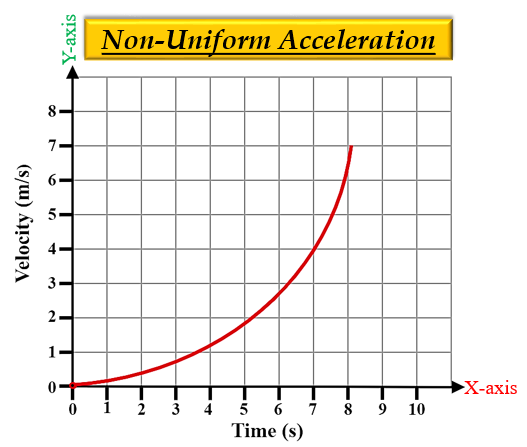# What is a constant acceleration and variable acceleration?

Acceleration is the measure of changing velocity, which implies how the speed of a body is changing at a particular time.

Uniform or constant acceleration is a type of motion in which the speed or velocity of an object changes by an equal amount in equal intervals of time.

For example- When a cyclist moving down the slope when he/she is not pedalling.

On a graph, it is represented on a velocity-time graph, and a straight line between them shows the uniform or constant acceleration.Non-uniform or variable acceleration is a type of motion in which the speed or velocity of an object changes by unequal amounts in equal interval of time.

For example- A bus moving on a crowded street.

On a graph, it is represented on a velocity-time graph, and a curved line between them shows the non-uniform or variable acceleration.